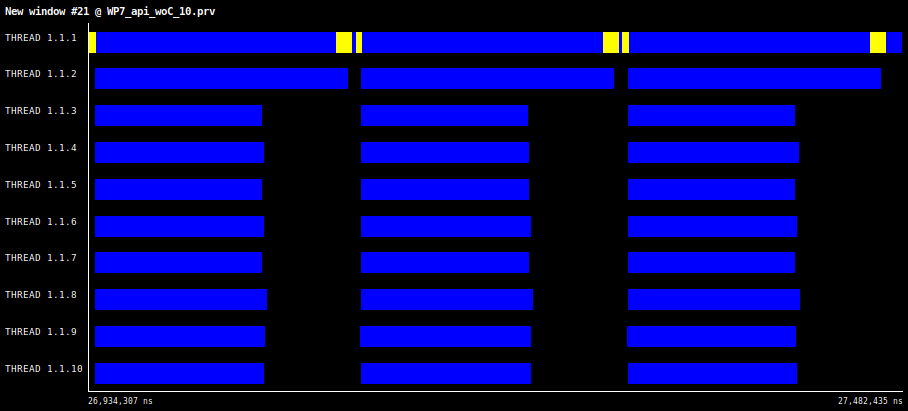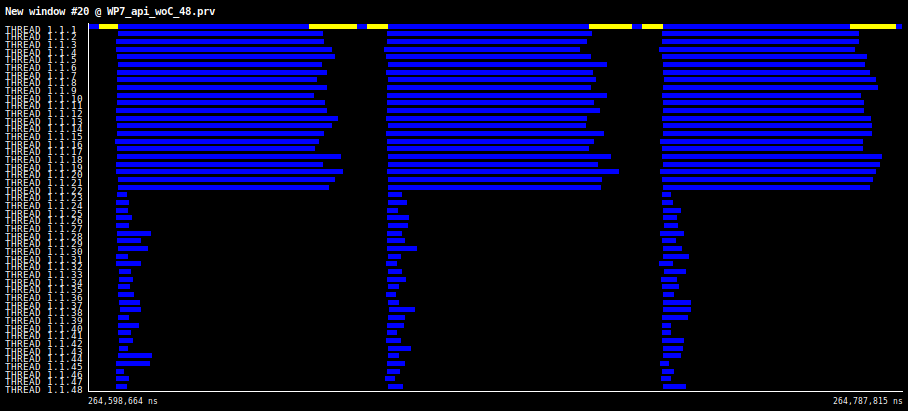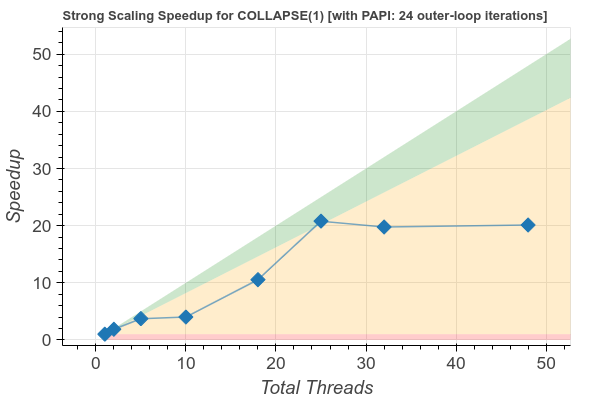# Lack of iterations on an OpenMP parallel loop

Usual symptom(s):
• Load Balance Efficiency: The Load Balance Efficiency (LBE) is computed as the ratio between Average Useful Computation Time (across all processes) and the Maximum Useful Computation time (also across all processes). (more...)

In some codes, the problem space is divided into a multi-dimensional grid on which the computations are performed. This pattern typically results in codes such as jCFD_Genesis, with multiple nested loops iterating over the grid, as shown in the following code snippet.

DO K = 1, Nk
DO J = 1, Nj
DO  I = 1, Ni
!! work to do
END DO
END DO
END DO


In the loop above, the work is related to computations from a real-case, where the results of multiplication and addition between elements of multiple three-dimensional matrices are stored in an output three-dimensional matrix of the same size.

When the computations on the grid are independent one from another it is possible to compute the values in parallel. In addition, there is often an outer loop iterating over multiple time steps. When implementing this pattern in parallel using OpenMP it is common to parallelize the loops as in the following code snippet, using a regular !$OMP PARALLEL DO directive. DO iter = 1, Niter !$OMP PARALLEL DO DEFAULT(NONE) SHARED(...)
DO K = 1, Nk
DO J = 1, Nj
DO  I = 1, Ni
!! work to do
END DO
END DO
END DO
!$OMP END PARALLEL DO END DO  However, poor load balance can occur because the number of iterations in the outer loop can’t be distributed evenly over the threads. Consider, for instance, the case where there are 22 K-loop iterations (Nk=22), and 21 threads. During execution of the 21th iteration, 20 of the 21 threads are idle. In Figure 1, an Extrae timeline window for three outer loop iterations with 10, 18 and 48 threads, is shown. The code executed inside the inner loop is the same for all values of I. As the number of threads increases the distribution of work becomes significantly imbalanced.Figure 1: Timeline view showing three outer iterations for a regular !$OMP PARALLEL DO nested loop with Nk=22, Nj=42, Ni=82, and Niter=24.

Figure 2 shows the speedup plot for Niter=24, Ni=492, Nk=22, and Nj=42, illustrating the impact of the imbalance when the number of threads exceed the number of iterations in the OpenMP loop.Figure 2: speedup plot for Niter=24, Ni=492, Nk=22, and Nj=42.

It can be observed that speed-up stalls when the number of threads exceed the number of parallelized iterations (i.e.: Nk=22).

Recommended best-practice(s):
Related program(s):
• OpenMP regular parallelization of nested loop